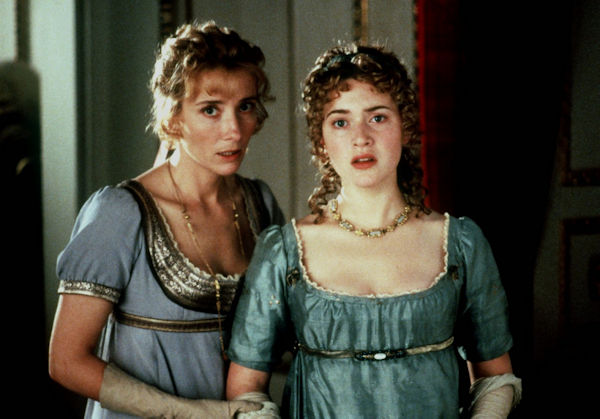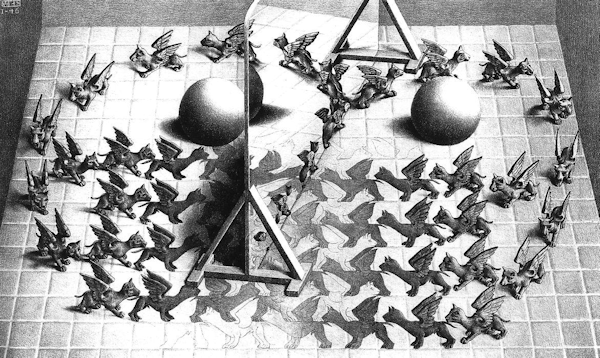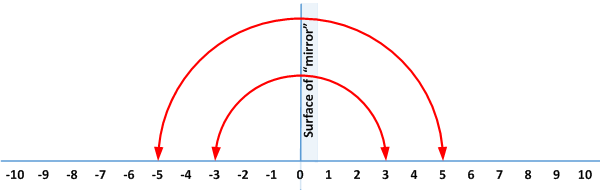# 4 – Rationality and Reality

 < ρℝεν | ℂσητεητs | ℕεχτ >“I could say the multiplication table up to six times seven is thirty-five, and I don’t reckon I could ever get any further than that if I was to live forever.” – Mark Twain, The Adventures of Huckleberry Finn

Multiplying the Multitude

The title of this chapter neither refers to a lost Jane Austen Novel, nor to an 18th century German philosophical treatise. Instead it emerges from considering something altogether more quotidian, namely multiplication. In Chapter 2 I defined what a Group is in Mathematical terms and the first examples I turned to in order to illustrate this concept all related to the addition of numbers . In the last Chapter, we moved on to cover some geometrical examples of Groups. Here I want to return to the numeric side of the house .

We previously showed that addition of numbers formed a Group, what about multiplication? Let’s start with the numbers we used last time, namely the integers. The set of integers is denoted by ℤ and consists of the following numbers: {… , -4, -3, -2, -1, 0, 1, 2, 3, 4, …}, where the ellipses  imply that the numbers continue indefinitely in both directions. We’ll define multiplication in the normal manner:

a × b = a + a + … + a (b times)

or symmetrically:

a × b = b + b + … + b (a times)

Let’s do our customary assessment of whether or not we have a Group.

1. Closure

Clearly if we multiply any two integers together we get another integer.

2. Identity

This time instead of 0, 1 seems to be the obvious candidate. For any integer a we have a × 1 = a.

3. Inverses

Here we run into two problems, one of these we can negotiate our way around, the other requires us to reassess our definition of the Group.

• 0 presents the first problem – there is no number a such that a × 0 = 1, because a × 0 = 0 for all numbers a. We can skirt around this complication by excluding 0 from our set. We could think about the set including non-zero positive and negative integers, {… , -4, -3, -2, -1, 1, 2, 3, 4, …}, sort of the integers with a hole in the middle. However, for our purposes, let’s consider just the non-zero positive integers, or equivalently the Natural Numbers, ℕ = {1, 2, 3, 4, …}.

• Having disposed of one problem, another rears its head. What is the inverse of 4 under multiplication? Well clearly it is a number a, which satisfies the equation:

a × 4 = 1.

Elementary algebra yields the solution:

a = ¼.

However ¼ is not a whole number and so definitively not a member of ℕ.

Divide and ConquerHere we’ll abandon our attempt to show that ℕ under multiplication forms a Group, it clearly doesn’t. What do we have to do in order to address this situation? The obvious answer is to admit numbers like ¼, what most people would refer to as fractions. What is a fraction? Well a fraction is one whole number divided by another whole number. So we might, if we again ignore numbers less than zero for the sake of simplicity, define the set of positive fractions as follows:

+ = {a / b, where a, b ∈ ℕ} 

This allows a whole range of numbers like ¼, but also:

2 / 3

22 / 7

24,798,622,896,734,982,164 / 349,72,349,823,678,568,237

and so on…

We call this set the positive Rational Numbers, if we include both zero and all negative fractions that we drop the + superscript and this set is denoted by a plain ℚ .

It is important to note two things:

1. The use of elements of ℕ to define our set avoids nastiness like dividing by zero.

2. + clearly also includes all elements of ℕ as these are just:

1 / 1

2 / 1

3 / 1

4 / 1

and so on…

Returning to our assessment of whether the positive Rational Numbers (now represented by ℚ+) form a Group under addition, the statements about Closure and an Identity still hold, let’s look at inverses again:

1. Inverses

We can now see that for any a and b ∈ ℕ:

(a / b) × (b / a) = (a × b) / (b × a) = 1

Or, using the customary notation of Mathematicians introduced in Chapter 2:

(a / b)(b / a) = ab / ba = 1

So we have inverses.

2. Associativity

It is a general property of multiplication that grouping things up in different ways makes no difference. So:

3 × (4 × 5) = 3 × 20 = 60

and

(3 × 4) × 5 = 12 × 5 = 60

This clearly still holds if we replace each of the Natural Numbers used above with fractions. So we have associativity.

This means that the positive Rational Numbers, ℚ+, do indeed form a Group under multiplication .

A Magic MirrorIn Chapter 2 we spoke about one type of symmetry evident in the integers under addition. This was that an element and its inverse were mirror images of each other. If the integers are represented by a line running left and right, then any integer’s inverse under addition is its reflection in a mirror running up and down and through the identity element, 0. This is shown below:We can make a similar observation about ℚ+ under multiplication, but one with some surprising properties.

First of all note that we can split ℚ+ into three subsets:

+big = {a / b, where a, b ∈ ℕ and a > b}

i.e. fractions bigger than 1

+1 = {a / b, where a, b ∈ ℕ and a = b}

i.e. all the different representations of 1, such as:

1 /1

204 / 204

and

349,72,349,823,678,568,237 / 349,72,349,823,678,568,237

and

+small = {a / b, where a, b ∈ ℕ and a < b}

i.e all fractions less than 1.

The combination of ℚ+big together with ℚ+1 and ℚ+small clearly covers all of ℚ+. Leaving ℚ+1 to one side for now, let’s think about the inverses of numbers in the other two sets.

For any number a / b in ℚ+big its inverse is clearly b / a. Because a > b this means that the inverse falls in ℚ+small.

Equally for any number a / b in ℚ+small its inverse is also clearly b / a. But because a < b this means that the inverse falls in ℚ+big.

Finally any number in ℚ+1 is equal to 1, which we have already established as our identity element and – as always – the inverse of the identity element is itself.

So we have shown a different symmetry. Here if our number line and mirror have the same orientation, then the mirror is placed at the identity 1. It is a rather odd mirror however. What it does is to reflect any member of ℚ+ to the right of 1 into a spot lying between 0 and 1. Equally, any Rational Number greater than 0 and less than 1 gets reflected to the line stretching rightwards from 1 to infinity. See below:We have constructed a magic mirror which can reflect a line of infinite length into a line of finite length, specifically with a length of 1. It can also reflect a line of length 1 into a line of infinite size. That’s a pretty amazing mirror and a rather non-intuitive form of symmetry. However, before I start to tread the well-worn path to the meaning of infinity, transfinite numbers and the Continuum Hypothesis, I’ll take a different route and leave this discussion for another day.

Expanding our HorizonsSo far in this Chapter we have expanded our definition of number from the Natural Numbers and Integers (ℕ and ℤ) to the Rational Numbers (ℚ). We did this by asking a question about whether numbers and multiplication formed a Group, a question firmly within the scope of this book. We asked for an answer to the equation:

What is the inverse under multiplication of 4?

In this section we are going to expand our definition of number further. Rather than having a specific Group Theoretical justification, I want to introduce a new set of numbers which we will need to use in later Chapters. These are the Real Numbers, denoted by ℝ. We’ll approach these by considering the solution to another question:

x2 = 2, what is x? 

The trite response is of course that the answer is √2 . But this is just shorthand for “number which when squared equals 2” so we have perhaps not advanced our cause so very far.

With a calculator (or spreadsheet) to hand, we can get an answer like:

√2 = 1.4142135623

However that equals sign is not entirely correct, what we have is an estimate of the value of √2 to ten decimal places . It can be rigorously demonstrated that the actual value of √2 extends to an infinite number of decimal places, or more prosaically the numbers to the right of the decimal point never stop .

Can we use fractions to represent √2? This is equivalent to saying that we can find two Natural Numbers a and b such that:

a / b = √2

or equivalently:

√2 ∈ ℚ .

There are various ways to show that there are no such numbers a and b. Each requires rather more Mathematics than I am looking to include in the main text. Interested readers are directed to the notes section . For those not intrepid enough to explore the proof provided, please take it on trust that we have come across a number which is not part of ℚ. This means that we need to extend our definition of number to capture this new exhibit.

We defined elements of ℚ as fractions of whole numbers (excluding zero as a denominator). How can we define this new set? Well we have come across one way already; we can define the set of Real Numbers, ℝ, as the set of all decimals expansions. This includes numbers like √2, as well as even more complicated numbers such as π ( 3.14159265358979323846…) and e (2.71828182845904523536…).

We can see that any member of ℚ is a member of ℝ by noting how accustomed we are to forming equations such as:

½ = 0.5

¼ = 0.25

⅓ = 0.33333… where the 3s go on to infinity

The last equation points to a way to discriminate between Real Numbers that are members of ℚ and those that are not.

The former will have decimal expansions that repeat themselves at some point.

Either ½ = 0.5000… or ½ = 0.499999… depending how pedantic you want to be

1 / 7 = 0.142857142857… where 142857 repeats itself for ever

The latter, like √2, will have decimal expansions that never settle down in this manner.

It can be shown that ℝ forms a Group under multiplication. However, again we must exclude 0. So using the set theory notation we defined in Chapter 2, the Group in question is (ℝ\0,×). The argument is precisely the the one used above to establish that ℚ+ is a Group (with the inverse of a Real Number x being 1/x), so I won’t repeat the same logic again.

As mentioned in Chapter 2, Mathematicians use a special symbol to show that something is a subset of another set (a set wholly contained in another set), this is ⊂. What we have shown so far with respect to numbers is:

ℕ ⊂ ℤ ⊂ ℚ ⊂ ℝ

It is of course tempting to consider whether this sequence can be extended further. One version of what might lie beyond ℝ is explored in Chapter 7. However in the next Chapter, we’ll introduce a way in which you can embed any of the number sets shown above into larger constructs, namely matrices.

 Concepts Introduced in this Chapter Rational Numbers Essentially fractions a / b where a is an integer and b is a non-zero integer. These are denoted by ℚ. Real Numbers An expansion of the concept of Rational Numbers to include all numbers which can be catalogued using an infinite (and possibly infinitely variable) decimal expansion, such as 3.141592… These are denoted by ℝ.
 Groups Discovered in this Chapter (ℚ+,×) The positive Rational Numbers under multiplication. (ℝ\0,×) The Real Numbers (excluding 0) under multiplication.
 < ρℝεν | ℂσητεητs | ℕεχτ >

Chapter 4 – Notes